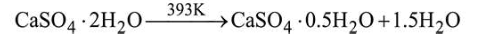# When gypsum is heated to 393 K,Question:

When gypsum is heated to $393 \mathrm{~K}$, it forms:

1. Anhydrous $\mathrm{CaSO}_{4}$

2. $\mathrm{CaSO}_{4} \cdot 5 \mathrm{H}_{2} \mathrm{O}$

3. $\mathrm{CaSO}_{4} \cdot 0.5 \mathrm{H}_{2} \mathrm{O}$

Correct Option: , 3

Solution:

Gypsum on heating to $393 \mathrm{~K}$ forms plaster of Paris.